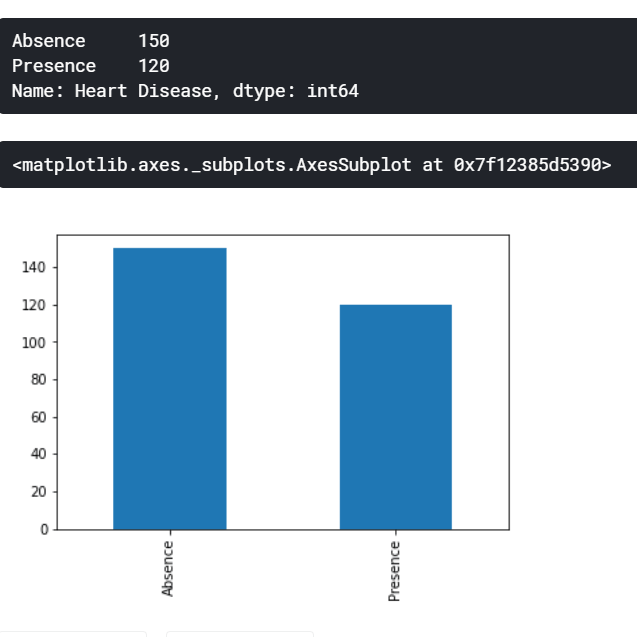# 如何在Python中使用Scikit-Learn进行特征选择？

2021年11月11日02:38:35 发表评论 1,076 次浏览

Python Scikit-Learn实现特征选择：了解如何使用 Python 中的 Scikit-Learn 库通过 SelectKBest、随机森林算法和递归特征消除 (RFE) 执行特征选择。

Python如何实现特征选择？在本文中，我们将重点介绍如何在我们的数据集上应用一些特征选择，这代表了数据预处理阶段的核心方面。但在深入编码和实现用于这些任务的不同技术之前，让我们首先定义特征选择的含义。

Python Scikit-Learn特征选择教程：我们将使用的数据集是来自 Kaggle心脏病预测数据集，你可以使用 Kaggle Kernel VM 直接处理该数据集，也可以将其下载到本地机器。

``\$ pip3 install numpy pandas matplotlib sklearn``

## 预处理

Python Scikit-Learn实现特征选择：我们将首先使用 Pandas 将我们的数据集加载到数据帧格式中。它由13 个特征加上标签组成，共有270行。

``````import numpy as np
import pandas as pd
import matplotlib.pyplot as plt
from sklearn.model_selection import train_test_split
from sklearn.preprocessing import MinMaxScaler
from sklearn.feature_selection import SelectKBest,chi2,RFE
from sklearn.ensemble import RandomForestClassifier

print(df.shape)

Python如何实现特征选择？上面的代码导入必要的库并从`data`文件夹中读取数据集 CSV 文件，接下来，你还需要创建`data`文件夹，你可以更改数据集文件在你的机器中的位置的路径。

``(270, 14)``

``````label = df["Heart Disease"]
df.drop("Heart Disease", axis=1, inplace=True)``````

``````print(label.value_counts())
label.value_counts().plot(kind="bar")````````````categorical_features = ["Sex", "Chest pain type", "FBS over 120", "EKG results", "Exercise angina", "Slope of ST", "Number of vessels fluro", "Thallium"]
df[categorical_features] = df[categorical_features].astype("category")``````

Python如何实现特征选择？我们现在将使用 来缩放我们的连续特征`MinMaxScaler`，它是一种归一化类型，其中值的范围在0到1之间，方程定义为`X_Norm = (X - X_Min) / (X_Max - X_Min).`

``````continuous_features = set(df.columns) - set(categorical_features)
scaler = MinMaxScaler()
df_norm = df.copy()
df_norm[list(continuous_features)] = scaler.fit_transform(df[list(continuous_features)])``````

## 使用卡方进行特征选择

Python Scikit-Learn实现特征选择：一卡方检验是用于统计测试两个事件的独立性。给定两个变量的数据，我们可以得到观察到的计数O和预期的计数E卡方测量预期计数E和观察计数O如何相互偏离。

• `k`：我们要选择的特征数量。
• `score_func`：选择过程所基于的功能。
``X_new = SelectKBest(k=5, score_func=chi2).fit_transform(df_norm, label)``

## 使用递归特征消除 (RFE) 进行特征选择

• `n_features_to_select`：我们要选择的特征数量。
• `estimator`：在每次迭代中将使用哪种类型的机器学习模型进行预测，同时递归搜索适当的特征集。
``````rfe = RFE(estimator=RandomForestClassifier(), n_features_to_select=5)
X_new = rfe.fit_transform(df_norm, label)
X_new``````

## 使用随机森林的特征选择

Python Scikit-Learn特征选择教程：基于树的机器学习算法`DecisionTreeClassifier`或它们的集成学习等价物`RandomForestClassifier`使用一组树，其中包含由分裂产生的节点。这些拆分的主要目的是通过使用诸如基尼指数之类的杂质度量来尽可能地减少杂质。这些基于树的模型可以通过计算该特征将导致的杂质减少量来计算该特征的重要性。

``````clf = RandomForestClassifier()
clf.fit(df_norm, label)
# create a figure to plot a bar, where x axis is features, and Y indicating the importance of each feature
plt.figure(figsize=(12,12))
plt.bar(df_norm.columns, clf.feature_importances_)
plt.xticks(rotation=45)``````

## Python Scikit-Learn实现特征选择总结

Python如何实现特征选择？此外，对于要选择的特征数量，这可以通过遵循迭代方法来回答，直到`k`(in `SelectKBest`) 收敛并且机器学习性能没有增加太多。

• 使用chi 2选择对标签依赖度高的特征。
• 使用 RFE 递归地找到给定估计量的最佳特征集。
• 使用随机森林等基于树的机器学习方法来显示有助于在拆分节点时尽可能减少杂质的特征。